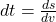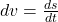## how you could calculate a time step (dt) if you have a vector of accumulative distance (ie the distance keeps adding every step) and an inst

Question

how you could calculate a time step (dt) if you have a vector of accumulative distance (ie the distance keeps adding every step) and an instantaneous velocity vector (ie the speed at that moment).

in progress 0
4 months 2021-07-29T13:56:28+00:00 2 Answers 1 views 0

dt = ds/dv.

Explanation:

to calculate the time step if you have a vector of accumulative distance and instanteneous velocity vector can be expressed bellow

solution

ds = dv/dt

divide the step distance by the instantaneous velocity you will get the time step.

that it

dt = ds/dv.

so to calculate the time step having a vector that keeps adding every step and speed at that moment is this dt = ds/dv.…………..Divide the accumulated distance by the instantaneous velocity

Explanation:

Let the accumulated distance be denoted as ds

Let the instantaneous velocity be given as dv

Using the relationship,If we make dt the subject of the formula:Therefore, this means that we can calculate the time step dt by dividing the accumulated distance by the instantaneous velocity.